XLL+ Class Library (7.0)

You can use the XLL+ Function Wizard to specify that an argument should contain a two-dimensional array - or matrix - of a particular type. This walkthrough demonstrates how to add and use a matrix argument in an add-in function.

The walkthrough also shows how to specify that a matrix input be square (i.e. have the same number of rows and columns).

You can find a copy of all the code used in this walkthrough in the `Walkthroughs/MatrixFns` folder in your XLL+ installation, along with a demonstration spreadsheet, `MatrixFns.xls`.

### Creating the project

#### To create the project using Visual Studio

1. From the File menu, select New and then Project to open the New Project dialog.
2. Select the XLL+ 7 Excel Add-in project template from the list of Visual C++ Projects, and enter MatrixFns in the Name box. Under Location, enter an appropriate directory in which to create the project.
3. Accept all the default settings in the XLL+ AppWizard.

### Creating the function

Use the XLL+ Function Wizard to create a new add-in function and to add a matrix argument.

Note: If you do not know how to start the Function Wizard, or you cannot find the command to open it, look at Invoke the Function Wizard in the User Guide.

1. Open the source file `MatrixFns.cpp` and click on the New XLL+ Function menu item or tool-button, to show the New Function dialog, and fill in the name as shown below.2. Name ExtractRow CXlOper Lookup & Reference Extracts a single row from a matrix
3. Fill in the function's category and description.

Add two new arguments by typing into the arguments grid, as shown below.

Type Name Description
Double[][] X Matrix whose row is to be extracted.
Unsigned Short Int Row 1-based index of row.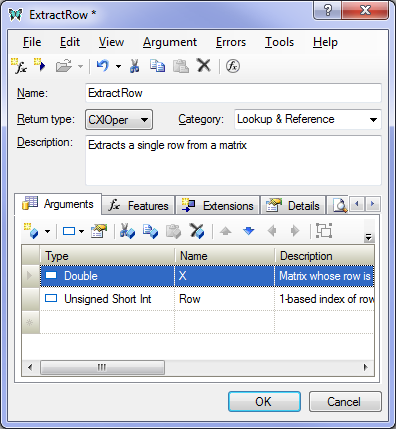4. Select the argument row containing `X`.

Click on the Matrix tool, to convert the argument to a matrix.5. The argument is now a matrix of type double, as shown below.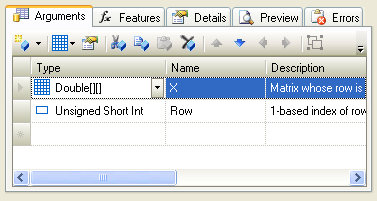6. Click on the OK button to close the Function Wizard and save the function.

### Inspecting the code

1. The following code has been added to `MatrixFns.cpp`.CopyC++
```CXlOper* ExtractRow_Impl(CXlOper& xloResult, const CXlOper* X_op, unsigned short
int Row)
{
// Input buffers
ple::mtx_ptrs<double> X;
// Validate and translate inputs
XLA_TRUNC_ONBLANK);
// End of generated code
//}}XLP_SRC
// TODO - set the value of xloResult, or return another value
//          using CXlOper::RetXXX() or throw a CXlRuntimeException.
return xloResult.Ret();
}```

Let us examine the interesting code.

2. Note that the matrix argument has been declared as type `const CXlOper*`. The CXlOper type is usually used to pass vector and matrix values from Excel to add-in functions.CopyC++
```CXlOper* ExtractRow_Impl(CXlOper& xloResult, const CXlOper* X_op, unsigned short
int Row)```
3. A buffer variable X is declared. The contents of the CXlOper argument passed by Excel will be extracted and put into X.

ple::mtx_ptrs<double> X;

The container class for the matrix is ple::mtx_ptrs.

Note: If you wish to use a different container class, for instance a matrix class that is used to pass values to your existing functions, you can use the XLL+ Function Wizard to change the container class.

4. Code has been generated to read the contents of `X_op` into `X`.

Note that if the cell range passed to the function cannot be read for any reason (for example, because a cell contains a string instead of a number) then an exception of type CXlConversionException will be thrown, input validation will cease, and a useful message will be returned to Excel.

### Completing the function

1. Add code to implement the function, as shown below:CopyC++
```CXlOper* ExtractRow_Impl(CXlOper& xloResult, const CXlOper* X_op, unsigned short
int Row)
{
// Input buffers
ple::mtx_ptrs<double> X;
// Validate and translate inputs
XLA_TRUNC_ONBLANK);
// End of generated code
//}}XLP_SRC

// Check the 1-based index
if (Row < 1 || Row > X.size(0))
throw CXlOutOfRangeException();
size_t r = (size_t)(Row - 1);

// Create a vector to receive the row
std::vector<double> extract;
for (size_t i = 0; i < X.size(1); i++)
extract.push_back(X[r][i]);

// Return the vector
xloResult = extract;
return xloResult.Ret();
}```
2. Note the use of the size() method to get the size of the matrix in each dimension.
3. Notice how the items in the matrix can be accessed exactly as if the matrix was an array.

extract.push_back(X[r][i]);

mtx_ptrs<T>::operator const T**() const

1. Build the project, using the Build/Build Solution menu or Shift+Ctrl+B keyboard short-cut. If you are working with a 64-bit version of Excel, then be sure to select the 64-bit platform `x64` before you build.
2. When the project has been built successfully, use the Debug/Start Debugging menu or F5 keyboard short-cut to run your add-in under Excel.
3. If Excel puts up any message boxes asking whether you trust the add-in, say yes.
4. Test the function by entering a matrix of numbers, and then using the `ExtractRow` function to extract one row, as shown below: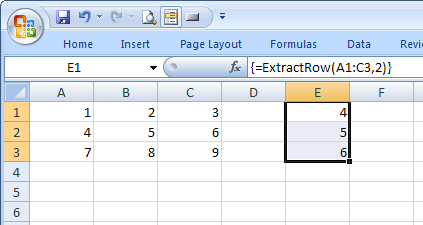Because `ExtractRow` is an array formula, you need to type Shift+Ctrl+Enter to enter it. Note the curly braces around the formula, indicating an array formula.

### Constraining the matrix to be square

You can use the XLL+ Function Wizard to constrain the input matrix to be square.

1. In Visual Studio, use the Tools/XLL+ Function Wizard menu to return to `ExtractRow`.

2. Select the row containing X and click on the Argument Details tool.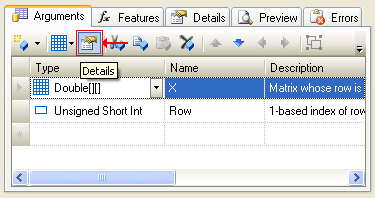3. Select the Matrix tab, and click on the drop-down button for Height is equal to. Select "widthOfX".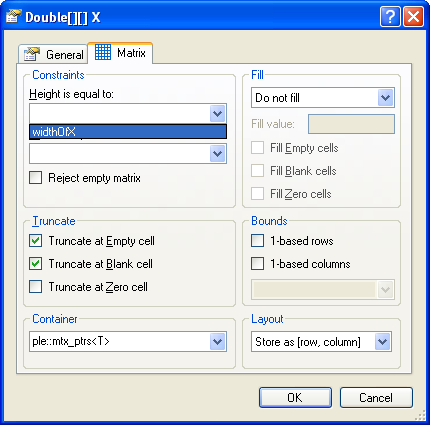4. Press OK to accept the changes to X, and the new constraint will be displayed in the Function Wizard.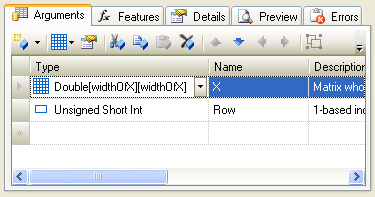#### Changes to the code

Examine the code in `MatrixFns.cpp`:CopyC++
```CXlOper* ExtractRow_Impl(CXlOper& xloResult, const CXlOper* X_op, unsigned short
int Row)
{
// Input buffers
ple::mtx_ptrs<double> X;
// Named bounds
long widthOfX = -1;
// Validate and translate inputs
XLA_TRUNC_ONBLANK, &widthOfX, 0, &widthOfX);
// End of generated code
//}}XLP_SRC

...
}```

A new local variable, `widthOfX` has been declared, with an initial value of -1. This is passed to `XlReadMatrix()` as the expected width of X and also as the expected height of X.

If the height of X is not found to be equal to its width then an exception of type CXlConversionException will be thrown, input validation will stop, and an error message will be returned to Excel, such as:

`#ERROR: Expected 4 columns for X`